## Plotting panning law graphStarted by 4 years ago10 replieslatest reply 4 years ago133 views

Hello, I am studying this code which uses the tangent law to locate a phantom image between two loudspeakers at 60º.

Tangent law:

tan(theta)/tan(alpha) = (g1-g2)/(g1+g2)

% Stereophonic panning example with tangent law
Fs=44100;
theta=30; % Virtual source
alpha=30; % Half of opening angle of loudspeaker pair (60º)
theta=theta/180*pi;
alpha=alpha/180*pi;
% Computing gain factors with tangent law
g(2)=1; % initial value has to be one
g(1)= -(tan(theta)-tan(alpha)) / (tan(theta)+tan(alpha)+eps);
% Normalizing the sum-of-squares
g=g/sqrt(sum(g.^2));
% Signal to be panned
signal=mod([1:20000]',200)/200;
% Actual panning
loudsp_sig=[signal*g(1) signal*g(2)];
% Play audio out with two loudspeakers
soundsc(loudsp_sig,Fs);

I am quite new to Octave/Matlab, does anyone know how I could plot the function g(theta)? I don't quite understand the equation used in g(1), since theta should be theta=arctan((g1-g2)*tan(alpha)/(g1+g2)), how could I plot theta using the latter?

[ - ]Hi Andresburgs.

Does MATLAB allow us to send two different signals to our left and right speakers? If so, I couldn't figure out how to do that.

I also do not understand your:

g(1)= -(tan(theta)-tan(alpha))/(tan(theta)+tan(alpha)+eps);

command. The Law of Tangents deals with the tangent of the difference between two angles. However, your above command involves the difference between two tangents. So the above command is a mystery to me, sorry.

Try this, it's mildly interesting:

clear g;

alpha = 30; % alpha in degrees

alpha = alpha*pi/180; % alpha in radians

for Loop = 1:360 % theta in degrees, one -to- 360 degrees

theta(Loop) = (Loop-180)*pi/180; % theta in radians (-pi -to- +pi)

g(Loop) = -(tan(theta)-tan(alpha))/(tan(theta)+tan(alpha)+eps);

end

figure(2), clf

plot(theta*180/pi, g, '-bs', 'markersize', 2),

ylabel('g'), xlabel('theta (deg.)'),

title(['alpha = ', num2str(alpha*180/pi), ' deg.']), grid on

[ - ]Thanks for your reply,I got this code from a book (DAFX), I'll link the chapter at the bottom.

I tried the code you proposed but still hasn't helped me figure out the solution.

I am expecting a plot with theta in 0-30º on the orizontal axis, and the normalized gain from 0-1 on the vertical axis, thus giving me the gain of one speaker at every point of theta[0,30], since the phantom image i.e. theta should be dependant of the gain.

ch5

[ - ]Hi Andresburgs.

 I looked at the "ch5" material you referenced. The authors throw down the:equation and call it the "tangent law" with *NO* explanation of its origin or derivation. One thing I did learn is that the above "tangent law" Eq. (1) has *NOTHING* to do with the traditional "Law of Tangents" in trigonometry. (So that answers one question that I had.)

 From my web searching it looks like the only place to find the derivation of the above Eq. (1) is:

J.C. Bennett, K. Barker, and F.O. Edeko, “A new approach to the assessment of stereophonic sound system performance,” J. Audio Eng. Soc., vol. 33,no. 5, pp. 314–321, May 1985.

 Ville Pulkki has written many papers giving Eq. (1), but, sadly, in none of his papers (that I could find) did he give the origin of that equation.

 I found five papers on the web discussing "stereophonic panning" and they all presented the above Eq. (1), also with *NO* explanation of its origin or derivation. I criticize those authors for this.

 At the web site:

I found the expression: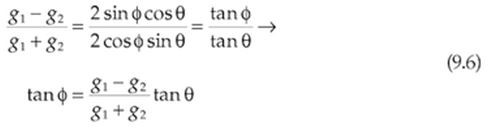but, again sadly, I did not have access to that material's previous pages to see a diagram that would help learn the origin of that Eq. (9.6). Equation (9.6) is enticing and I wish I could learn its derivation. (After reviewing Trigonometry's "function-product identities" I was still unable to make sense of Eq. (9.6).)

 While I am griping here, I say shame on the "ch5" material's authors for giving their weird MATLAB command

g(1)=- (tan(theta)-tan(lsbase)) / (tan(theta)+tan(lsbase)+eps);

with no explanation whatsoever!

 I'm sorry I could not be of much help to you.

[ - ]Hi andresburgs.

Are you still working on your "panning law graphing" problem?

By the way, this evening I developed a derivation for the following equation.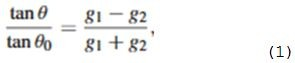[ - ]Hi Rick, thanks for your help.

Yes I am still working on this project.

I tried from scratch using this code:

phi = 30;
g1 = 1;
g2 = [0:0.1:1];
theta = atand((tand(phi))*((g1-g2)./(g1+g2)));
plot(theta, g2)

Which gives me  the plot of theta in function of g, but I am not sure if this is the right way to do it.

Would love to hear about the derivation you developed.

[ - ]Hi andresburgs.

In you code, you should make a change so that as g2 increases in value, g1 must simultaneously decrease in value. We must ensure that g1 squared plus g2 squared always equals one. Based on the following diagram: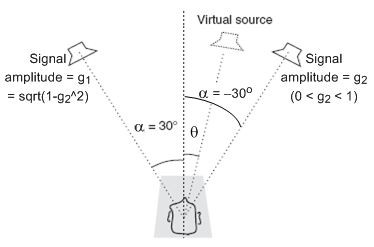I have MATLAB code that generates the following curve: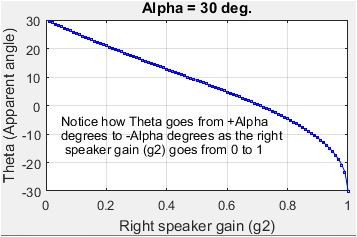As the right speaker gain g2 goes from 0 -to- 1, left speaker gain g1 goes from 1 -to- 0.
[ - ]How did you obtain that curve?

[ - ]Hi. Here's the MATLAB code I used to plot the above curve in my earlier Feb. 16, 2019 post.

% Filename: Stereophonic_panning.m
%
% Stereophonic panning example using "stereophonic tangent law".
% Finds the apparent angular direction (Theta) of a common sound being
% radiated by two symmetrically placed speakers. Left speaker signal
% amplitude is 'g1', and right speaker signal amplitude = 'g2'.
%
% The "stereophonic tangent law" states that:
%
%                  tan(Alpha)(g1 -g2)
%   tan(Theta) =  ---------------------
%                       g1 + g2
%
% [Richard (Rick) Lyons, Feb. 16, 2019]

clear, clc
Alpha = 30; % Half of opening angle of loudspeaker pair (60º)
Alpha = Alpha/180*pi; % Alpha in radians
Tan_Alpha = tan(Alpha);

% Vary the g2 amplitude of right speaker, 0.01 < g2 < 1, and compute Theta
for K = 1:100
g2(K) = K/100; % 0.01 < g2 < 1.0

% Compute g1 based on g2 amplitude
g1(K) = sqrt(1 -g2(K)^2); % Sum of radiated powers always equals one.
% Find Theta using "stereophonic tangent law"
Theta(K) = atan2(Tan_Alpha*(g1(K)-g2(K)),(g1(K)+g2(K)));
end
Theta = Theta*180/pi; % Theta in degrees

% Plot Theta (phantom speaker angle angle) vs gain g2
figure(1)
plot(g2, Theta, '-bs', 'markersize', 2)
xlabel('Right speaker gain (g2)'), ylabel('Theta (Apparent angle)')
title(['Alpha = ', num2str(Alpha*180/pi), ' deg.'])
text(0.05, -5, 'Notice how Theta goes from +Alpha')
text(0.05, -10, 'degrees to -Alpha degrees as the right')
text(0.05, -15, ' speaker gain (g2) goes from 0 to 1')
grid on, zoom on

[ - ]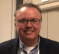Does MATLAB allow us to send two different signals to our left and right speakers?

Not sure if this was answered below.  The MATLAB sound() and soundsc() commands should produce stereo output if the underlying computer system/hardware supports that operation.  The data argument is an Nx2 matrix in that case.

Alternatively, the data could be written from MATLAB as a WAV file and an external system() call be used to belch out the sound.

[ - ]